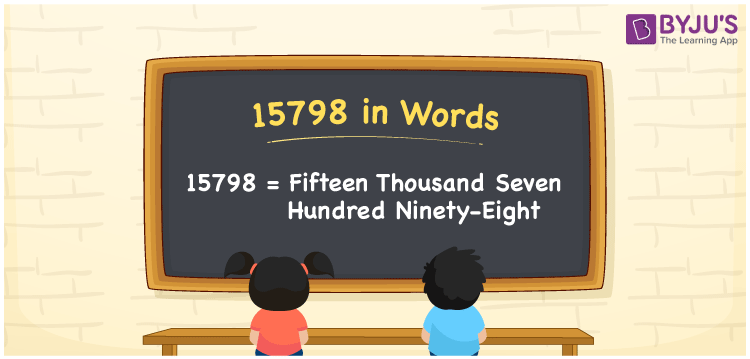# 15798 in Words

15798 in words is written as Fifteen thousand seven hundred ninety-eight. In both the International System of Numerals and the Indian System of Numerals, 15798 is written as Fifteen thousand seven hundred ninety-eight. The number 15798 is a Cardinal Number as it denotes some quantity. For example, “that mobile phone costs 15798 rupees”.

 15798 in Words Fifteen thousand seven hundred ninety-eight Fifteen thousand seven hundred ninety-eight in Number 15798

## 15798 in English Words

15798 in English words is read as “Fifteen thousand seven hundred ninety-eight”.## How to Write 15798 in Words?

To write 15798 in words, we shall use the place value chart. In the place value chart, put 1 in the ten thousands, 5 in the thousands, 7 in the hundreds, 9 in the tens and 8 in the ones. Let us make a place value chart to write the number 15798 in words.

 Ten Thousands Thousands Hundreds Tens Ones 1 5 7 9 8

Thus, we can write the expanded form as

1 × Ten Thousand + 5 × Thousand + 7 × Hundred + 9 × Ten + 8 × One

= 1 × 10000 + 5 × 1000 + 7 × 100 + 9 × 10 + 8 × 1

= 10000 + 5000 + 700 +90 + 8

= 15798

= Fifteen thousand seven hundred ninety-eight.

15798 is a natural number, the successor of 15797 and the predecessor of 15799.

15798 in words – Fifteen thousand seven hundred ninety-eight

• Is 15798 an odd number? – No
• Is 15798 an even number? – Yes
• Is 15798 a perfect square number? – No
• Is 15798 a perfect cube number? – No
• Is 15798 a prime number? – No
• Is 15798 a composite number? – Yes

## Frequently Asked Questions on 15798 in Words

Q1

### How to write 15798 in words?

15798 in words is written as Fifteen thousand seven hundred ninety-eight.
Q2

### How to write 15798 in the International and Indian System of Numerals?

In both, the system of numerals, 15798 in words, is written as Fifteen thousand seven hundred ninety-eight.
Q3

### How to write 15798 in a place value chart?

In the place value chart, write 1 in the ten thousands, 5 in the thousands, 7 in the hundreds, 9 in the tens and 8 in the ones, respectively.